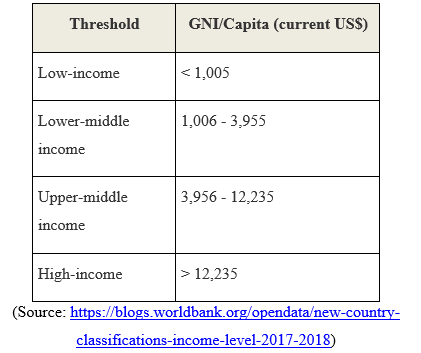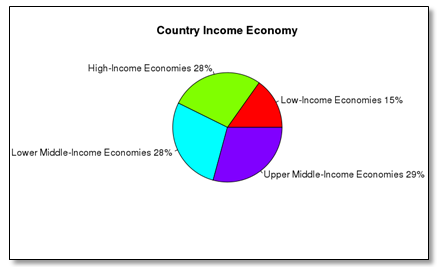## Practical Activity: Big Data Ecosystem

• Perform the installation of RHadoop's rmr and rhdfs packages as in section 5.9.A.

• Run the examples on squaring the numbers 1 to 100, firstly using a simple R script, and, secondly using the MapReduce function of the RHadoop rmr package. In the console, observe the number of splits and the mapping and reducing processes.

• You will now perform Data Analysis using RHadoop

• Run the example in section 5.9.C and see the generated pie chart.

• Make a copy of the above script given for MapReduce function. Modify the new script so that it uses the MapReduce function to determine the percentage of countries falling under each of the following classification:• The expected outputs should be as follows:

$key GNI 1 "Low-Income Economies" 5 "High-Income Economies" 4 "Lower Middle-Income Economies" 2 "Upper Middle-Income Economies"$val

 27 49 50 52